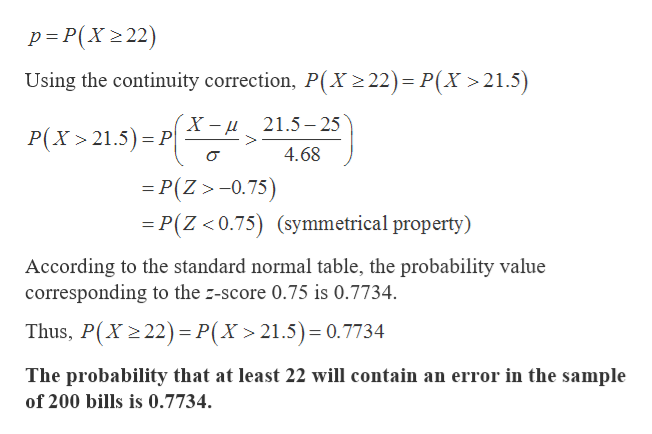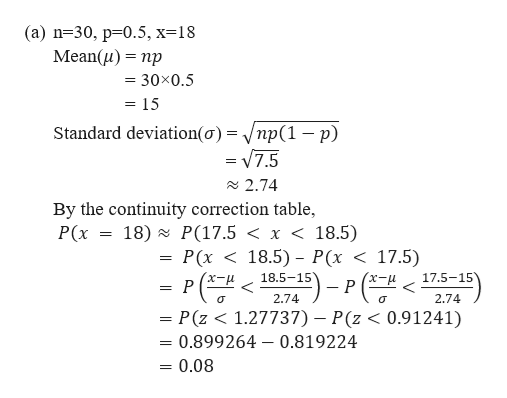# About 12.5% of restaurant bills are incorrect. If 200 bills are selected at random, find the probability that atleast 22 will contain an error. Is this likely or unlikely to occur? Use the normal approximation to the binomial to find the probabilities for the specific value(s) of X. aA. n=30, p=0.5, x=18B. n=50, p=0.8, x=44C. n=100, p=0.1, x=12

Question

About 12.5% of restaurant bills are incorrect. If 200 bills are selected at random, find the probability that atleast 22 will contain an error. Is this likely or unlikely to occur?

Use the normal approximation to the binomial to find the probabilities for the specific value(s) of X. a

A. n=30, p=0.5, x=18

B. n=50, p=0.8, x=44

C. n=100, p=0.1, x=12

check_circleExpert Solution
Step 1

It is given that 12.5% of restaurant bills contain error. So, the proportion of bills contain error (p) is 0.125.

Here, the sample size (n) is 200.

Let X be the random variable that is defined as the number of bills containing error which follows binomial distribution with n=200 and p=0.125.

Using normal approximation to the binomial distribution, follows normal distribution with,

Step 2

The probability that at least 22 will contain an error can be calculated as follows:help_outlineImage Transcriptionclosep P(X2 22) Using the continuity correction, P(X 2 22)= P(X >21.5) X -и P(X 21.5) P 21.5-25 4.68 =P(Z>-0.75) P(Z <0.75) (symmetrical property) According to the standard normal table, the probability value corresponding to the z-score 0.75 is 0.7734. Thus, P(X 2 22) P(X > 21.5)= 0.7734 The probability that at least 22 will contain an error in the sample of 200 bills is 0.7734. fullscreen
Step 3

Now the second part of the question is solved by using the normal approximation ...help_outlineImage Transcriptionclose(а) n%330, р30.5, х%318 Mean(u) np 30x0.5 = 15 Standard deviation(o) = /np(1 -p) = V7.5 2.74 By the continuity correction table, Р(x 18) P(17.5 < x < 18.5) Р(х < 18.5) - Р(< < 17.5) 18.5-15 х-и P 17.5-15 - P (- 2.74 2.74 = P(z < 1.27737) - P(z < 0.91241) = 0.899264 0.819224 0.08 fullscreen

### Want to see the full answer?

See Solution

#### Want to see this answer and more?

Solutions are written by subject experts who are available 24/7. Questions are typically answered within 1 hour*

See Solution
*Response times may vary by subject and question
Tagged in

### Probability